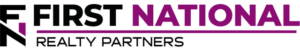# Understanding the Difference Between IRR and XIRR In Commercial Real Estate

### Key Takeaways

• To evaluate the performance of a commercial real estate investment, one commonly used metric is called the Internal Rate of Return or “IRR.”
• Conceptually, IRR is the discount rate that sets the net present value of all future cash flows equal to zero.  It is calculated using the relevant functions in Microsoft Excel and it requires a series of cash flows as inputs.

When evaluating the performance of a commercial real estate investment, there are a number of metrics that are traditionally used to measure returns.  One of the most common is called the internal rate of return.

By definition, the Internal Rate of Return (IRR) is the discount rate that sets the Net Present Value of a series of cash flows equal to zero.  But, just because the NPV is zero does not mean it is a bad investment, it just means that an investor will earn the IRR as their rate of return.

In order to calculate the IRR, it is first necessary to estimate a series of cash flows that an investor expects to earn.  This is done by creating a pro forma projection of a property’s income, expenses, and debt service.  If the property is profitable, there is money left after the loan payments have been made, which is available to be distributed to investors.  These are the cash flows that are used as inputs in the IRR calculation.

In this article we’ll discuss how to calculate IRR and the differences between XIRR vs. IRR.

## How to Calculate The Internal Rate of Return (IRR)

The formula used to calculate IRR is complex.  Fortunately, the calculation is made easier by using a function in Microsoft Excel or similar spreadsheet program.  The required inputs for the IRR function are simply the estimated pro forma cash flows, including a “year 0” cash flow that represents the initial investment in the property (a negative value).  For example, assume that the following series of cash flows are projected for a real estate investment: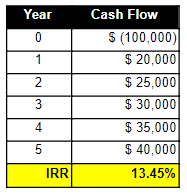Using these cash flows, the IRR function inputs would be “=IRR(-\$100,000, \$20,000, \$25,000, \$30,000, \$35,000, \$40,000).  The resulting IRR is calculated as 13.45%, which is a solid annual return.

However, the IRR function can be misleading when calculating commercial real estate investment returns.  This function is designed to assume that the time period between all cash flows is equal.  This is unrealistic because it is unlikely that the initial outflow for a property will be on the first day of the year and that each successive cash flow will be at the exact same interval in the future.

In reality, it is much more likely that a property will be purchased on any given day in the year and that the cash flows will occur at irregular intervals.  For these reasons, the “XIRR” function is the better measure of return for irregular cash flows.

## Calculating Internal Rate of Return using the XIRR Function

The major difference between the IRR formula and the XIRR formula is that XIRR uses the dates of the future cash flows and incorporates them into the return value by calculating the exact number of days between each period.  A few days here and there may seem insignificant, but the difference can be significant.

To illustrate this point about XIRR, consider the same series of periodic cash flows.  Now, assume that the initial outlay for the purchase (year 0) occurs on June 30.  The first cash inflow occurs on 12/31 of the same year and the successive annual cash flows also occur on 12/31 of the following years.  They are displayed in the following table: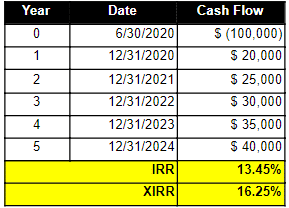Again, the XIRR function incorporates both the dates and the amount of the cash flows.  In Excel, the input would be like this:  =XIRR(Dates, Cash Flows).  The result of this calculation is 16.25%, which is a significant difference from the result using the IRR function.

## XIRR vs. IRR – Why The Difference Matters

Many commercial real estate investments offer a “waterfall” return distribution, meaning the cash flow split between the transaction sponsor and the investors is based on a series of return “hurdles.”  In many cases, the hurdle rate is calculated using the Internal Rate of Return.  For example, a typical 3-tier waterfall could look something like this:

• Tier 1:  If the property earns an IRR of 0% – 8%, the investors get 90% of the cash flow and the sponsor gets 10%.
• Tier 2:  If the property earns an IRR of 8% – 14%, the investors get 80% of the cash flow and the sponsor gets 20%.
• Tier 3:  If the property earns an IRR of 14% or above, the investors get 70% of the cash flow and the sponsor gets 30%.

In such a structure, the investors get 80% of the cash flow when the Internal Rate of Return is calculated using the IRR function, which assumes regular intervals between cash flow iterations.

However, the result of the XIRR calculation bumps the cash flow split to the next tier where the investors get 70% of the cash flow.

The point is, the difference between the results of the XIRR vs. IRR calculations can impact the cash flow split in a waterfall return structure.  As such, it is critically important that investors read all of the offering materials to determine how IRR is calculated and which function is used.

## Interested in Learning More?

First National Realty Partners is one of the country’s leading private equity commercial real estate investment firms. With an intentional focus on finding world-class, multi-tenanted assets well below intrinsic value, we seek to create superior long-term, risk-adjusted returns for our investors while creating strong economic assets for the communities we invest in.

Get instant access to all of our current and past commercial real estate deals.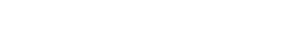## Subscribe Now

Get the latest news on real estate

1031 Exchange

Acquisitions

CRE Basics

## Get More From FNRP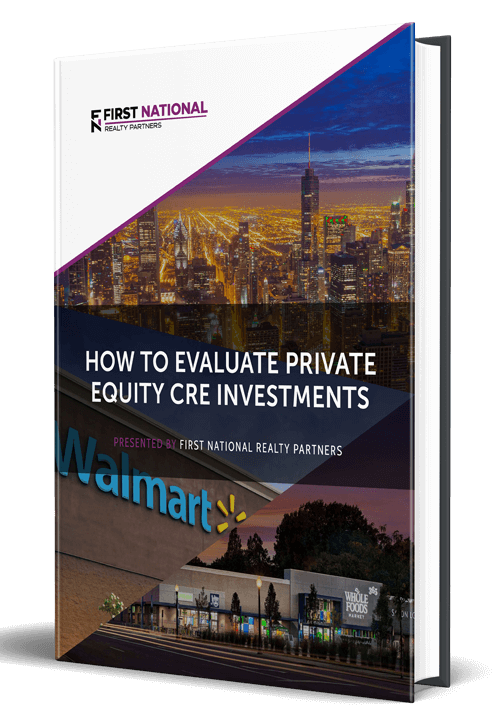## How to Evaluate Private Equity CRE Investments## How to Complete a 1031 Exchange with a Private Equity Sponsor